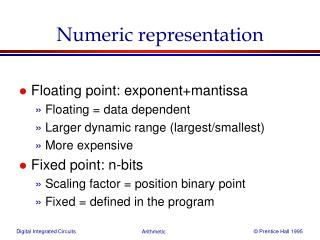DownloadDownload PresentationNumeric representation

# Numeric representation

Download Presentation## Numeric representation

- - - - - - - - - - - - - - - - - - - - - - - - - - - E N D - - - - - - - - - - - - - - - - - - - - - - - - - - -
##### Presentation Transcript

1. Numeric representation • Floating point: exponent+mantissa • Floating = data dependent • Larger dynamic range (largest/smallest) • More expensive • Fixed point: n-bits • Scaling factor = position binary point • Fixed = defined in the program

2. Numeric representation

3. Numeric representation • One’s complement • Negative num = bit_inverse(positive num) • Two’s complement • Negative num = bit_inverse(positive num) + 1 • Conversion to more bits via sign extension • E.g. 6 = 011 = 0011 = 00011 … • E.g. –6 = 101 = 1101 = 11101 … • Temporary overflow 1 0 1 1 1 -24 23 22 21 20

4. Temporary overflow

5. Integer, fractional and scaling Integer Fractional 1 0 1 1 1 1 0 1 1 1 Binary point -20 2-1 2-2 2-3 -24 23 22 21 20 2-4 -2(n-1)  x  2(n-1) -1 range -1 x  1-2-(n-1) Scaling is chosing dependent on the signal range

6. Numeric representation: scaling • integer and fractional numbers are a special case of fixed point • fix <p,q> (ART designer & SystemC) p q 1 0 1 -19/8 = -2.375 1 1 1 fix <8,3> 1 0 -24 23 22 2-2 21 20 2-1 2-3 Scale factor 1/8 negative weight 2’s complement quantization error Same alu handles fix <8,1>, fix <8,2>, fix <8,3>, ... if q=0 then integer e.g. int <8,0> if q=p-1 then fractional e.g. int <8,7>

7. Fixed point: addition -19/8 Int <8,3> 1 1 1 0 1 1 1 0 Int <8,4> 97/16 1 0 1 0 0 1 0 0 59/16 0 0 1 1 1 0 1 0 1

8. Fixed point: multiplication -19/8 1 1 1 0 1 1 Int <8,3> 1 0 Int <8,4> 97/16 1 0 1 0 0 1 0 0 -1843/128 1 1 1 0 1 1 1 0 0 0 1 1 1 0 1 0

9. Arithmetic Building Blocks

10. A Generic Digital Processor

11. Building Blocks for Digital Architectures Arithmetic unit Bit-sliced datapath adder - ( , multiplier, shifter, comparator, etc.) Memory - RAM, ROM, Buffers, Shift registers Control - Finite state machine (PLA, random logic.) - Counters Interconnect - Switches - Arbiters - Bus

12. Bit-Sliced Design

15. Express Sum and Carry as a function of P, G, D

17. Complimentary Static CMOS Full Adder

18. Inversion Property

19. Minimize Critical Path by Reducing Inverting Stages

20. The better structure: the Mirror Adder

21. The Mirror Adder • The NMOS and PMOS chains are completely symmetrical. This guarantees identical rising and falling transitions if the NMOS and PMOS devices are properly sized. A maximum of two series transistors can be observed in the carry-generation circuitry. • When laying out the cell, the most critical issue is the minimization of the capacitance at node Co. The reduction of the diffusion capacitances is particularly important. • The capacitance at node Co is composed of four diffusion capacitances, two internal gate capacitances, and six gate capacitances in the connecting adder cell . • The transistors connected to Ci are placed closest to the output. • Only the transistors in the carry stage have to be optimized for optimal speed. All transistors in the sum stage can be minimal size.

23. NMOS-Only Pass Transistor Logic

25. NP-CMOS Adder C o1 S 1 A 1 B 1 S 0 A 0 B 0 C i0

26. Manchester Carry Chain

27. Sizing Manchester Carry Chain

29. Manchester-Carry Implementation

31. Carry Ripple versus Carry Bypass

33. Carry Select Adder: Critical Path

34. Linear Carry Select

35. Square Root Carry Select

41. The Binary Multiplication

42. The Binary Multiplication

43. The Array Multiplier

44. The MxN Array Multiplier— Critical Path Critical Path 1 & 2

45. Carry-Save Multiplier

46. Adder Cells in Array Multiplier

47. Multiplier Floorplan

48. Wallace-Tree Multiplier

49. Multipliers —Summary

50. The Binary Shifter